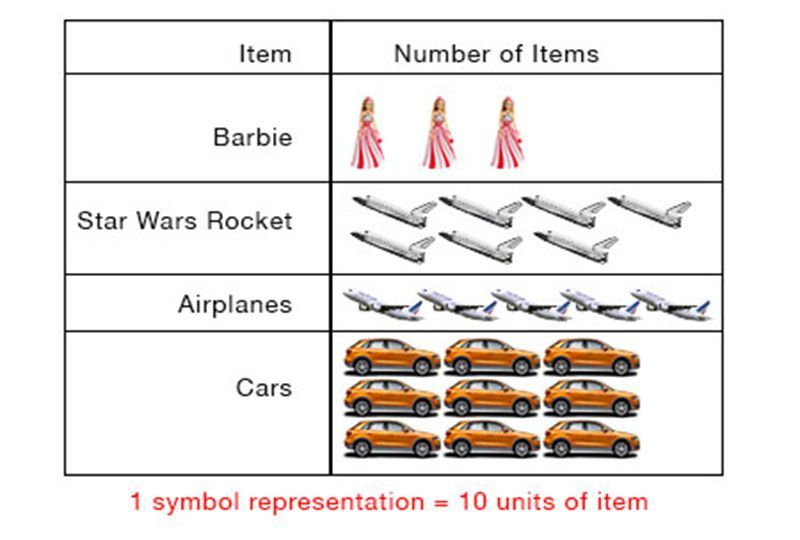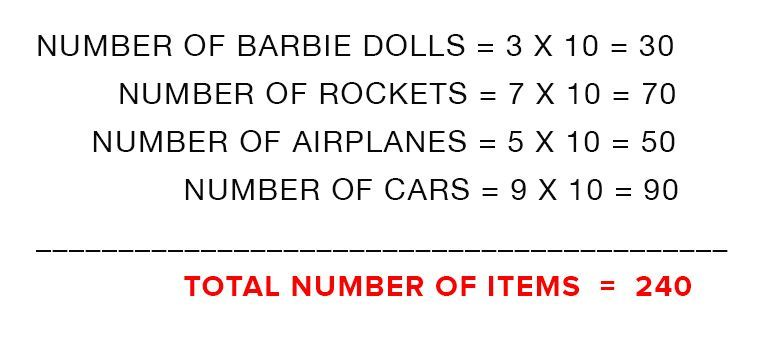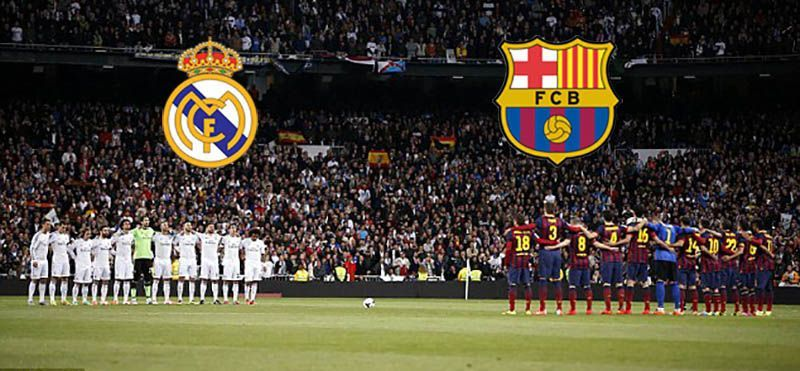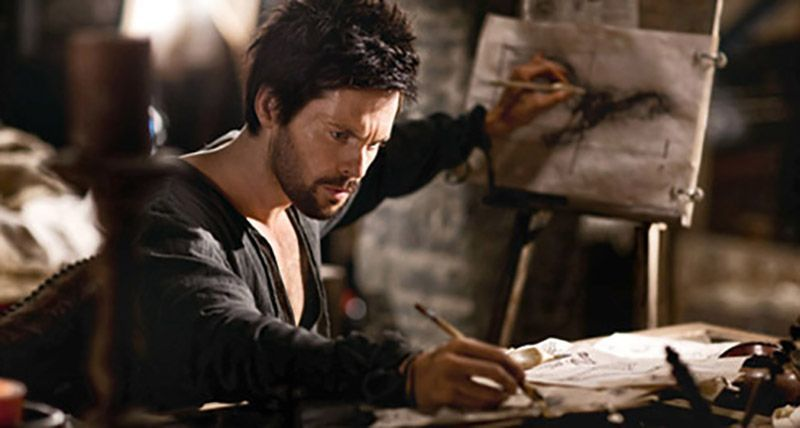# The Pictograph.

A pictorial representation, which show the type of quantity and number of each quantity is called a pictograph.This pictograph represents the different toys available in the section of the supermarket.

And the symbol is a representation of the number of toys of each type. E.g. number of cars is 9 symbols.

The number at the base of the pictograph represents the number of items represented by one symbol in the diagram.

The total number of items of each type is equal:

Number of Symbols x Number of items represented by symbol.

# Solved Example

How many items are there in the whole section in total?Solution:# Access All Content

## More from Grade 4 Math# Pictograph

Representing your world in pictures! See how the type and number of a quantity can be mathematically represented pictorially.# Venn Diagram

The easiest way to imagine sets visually. See the power of Venn Diagrams.# Venn Diagram Example

Learn how to use Venn Diagrams to solve real world problems with these interesting examples.